Importance: High ✭✭✭
 Author(s):
 Subject: Graph Theory » Extremal Graph Theory
 Keywords: graph packing
 Posted by: asp on: March 23rd, 2013
Conjecture  (BEC-conjecture)   If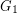andare-vertex graphs and, thenandpack.

A pair of-vertex graphsandare said toif they are edge-disjoint subgraphs of the complete graph onvertices.

The main conjecture in the area of graph packing is the abovementioned conjecture by Bollobás, Eldridge [BE] and Catlin [C].

In support of the BEC-conjecture, Sauer and Spencer [SS] proved that ifandare-vertex graphs andthenandpack.

Given a graph,denotes the line graph ofand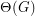denotes the number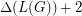. Kostochka and Yu [KY1] proved that ifandare two-vertex graphs with, thenandpack with the following exceptions: (1)is a perfect matching andis eitherwithodd or contains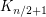or (2)is a perfect matching andiswithodd or contains.

Kostachka and Yu [KY2] conjectured that ifandare-vertex graphs with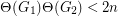thenandpack.

## Bibliography

*[BE] B. Bollabás and S. E. Eldridge, Maximal matchings in graphs with given maximal and minimal degrees, Congr. Numer. XV (1976), 165--168.

*[C] P. A. Catlin, Embedding subgraphs and coloring graphs under extremal degree conditions, Ph. D. Thesis, Ohio State Univ., Columbus (1976).

[KY1] A. V. Kostochka and G. Yu, An Ore-type analogue of the Sauer-Spencer Theorem, Graphs Combin. 23 (2007), 419--424.

[KY2] A. V. Kostochka and G. Yu, An Ore-type graph packing problems, Combin. Probab. Comput. 16 (2007), 167--169.

[SS] N. Sauer and J. Spencer, Edge disjoint placement of graphs, J. Combin. Theory Ser. B 25 (1978), 295--302.

* indicates original appearance(s) of problem.